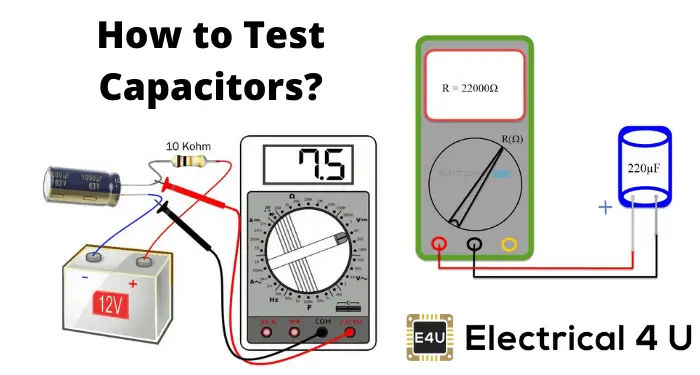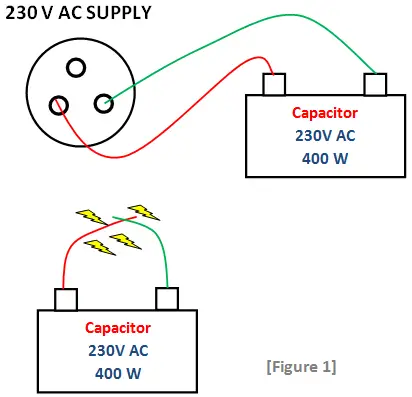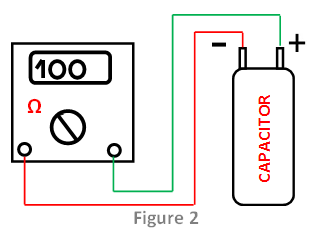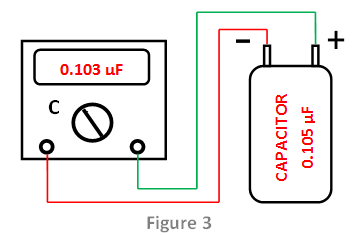# How To Test A Capacitor (Using a Multimeter, Voltmeter or AC Supply)

ContentsCapacitors are charge storing devices that form part of most electrical and electronic circuits.

Capacitors need to be checked and tested during servicing or troubleshooting.

There are a few different methods for testing a capacitor:

• Using an AC Supply (Not Recommended)
• By Using the Ohmmeter Setting Mode of a Multimeter
• By Using the Capacitance Setting Mode of a Multimeter
• By Using a Voltmeter

## Traditional Method to Test Capacitors

This method is really dangerous and is recommended only for professional Electrical engineers. For this method, it is safe to use 24V DC or 220-224V AC than 230V AC.

If 220-224V AC is used, series resistors should be connected in between the capacitor.The procedure is as follows:

1. The capacitor to be tested should be disconnected or a minimum of one lead of the suspected capacitor should be disconnected.
2. The capacitor should completely in discharge condition.
3. The leads should be connected to the terminal of the capacitor.
4. Next, these leads should be connected for a very short time (1- 4 seconds) to a 230V AC supply.
5. Now, remove the leads from the supply.
6. This is the important and most dangerous step. Short the terminals of the capacitor.
7. We can see that when the capacitor is good, a strong spark is produced.
8. When the capacitor is bad or not in good condition, a weak spark is produced.

## How to Test a Capacitor With a Multimeter

### By Using The Ohmmeter Setting Mode of a Multimeter

This method is used for finding the resistance of the capacitor and finding the condition of the capacitor (Figure 2).The procedure is as follows:

1. The capacitor to be checked must have to be in discharged condition.
2. The multimeter should be in an ohmmeter setting.
3. Now, connect the leads of the meter to the terminals of the capacitor.
4. The reading should be noted and the result should be compared with the following.
5. The low resistance value indicates a short capacitor.
6. If there is no deflection in the meter; that means, it is an open capacitor.
7. When there is a condition that at first the meter shows a low resistance and then it increases progressively to infinite, then it is a good capacitor.

### By Using The Capacitance Setting Mode of a Multimeter

This is the simplest and excellent method for checking the capacitor (Figure 3).The procedure is as follows:

1. The capacitor to be checked must have to be in discharged condition.
2. The multimeter should be in a capacitance setting.
3. Now, connect the leads of the meter to the terminals of the capacitor. The polarity is not a concern here.
4. The capacitance value which is almost near the capacitance rating of the suspected capacitor is shown in the meter.
5. If the capacitor is defective, we can see a lower value of capacitance or no values in the meter.

## How To Use a Voltmeter to Test a Capacitor

This method is used to find the potential difference across the capacitor. The procedure is as follows:

1. The capacitor is first charged with DC voltage which is less than the capacitor rating.
2. The polarity is important here. The longer lead (anode) of the capacitor to be connected to a positive voltage and the shorter lead (cathode) is connected to the ground or negative terminal of voltage.
3. The terminals of the voltmeter should be connected across the terminals of the capacitor.
4. When it reads the same voltage as we charged and afterward declines, then the capacitor is in good condition.
5. When the capacitor is not getting charged and the voltmeter shows reading, then the capacitor is not good or in defective condition.

The above four tests are implemented for finding the capacitor effectiveness. That is to determine the condition of a capacitor.

Want To Learn Faster? 🎓
Get electrical articles delivered to your inbox every week.
No credit card required—it’s 100% free.1.